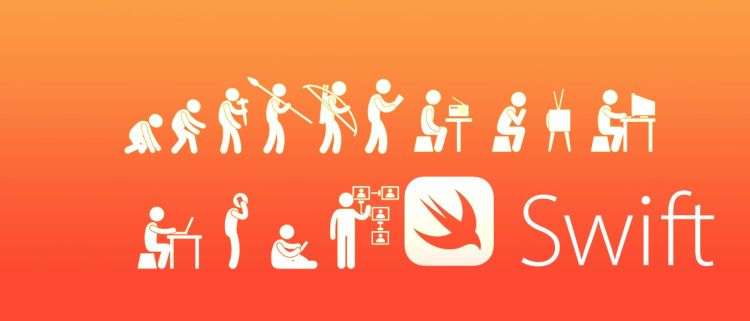← back to the blog

# How looks like a tuple in Swift? What is touple? Touples and Swift 5.1

##### Posted on November 19th, 2019 in Swift by GeorgeA function that takes and array of integers and returns a touple.

``````func calcStatistics(scores: [Int]) -> (min: Int, max: Int, sum: Int)
{
var min = scores;
var max = scores;
var sum = 0;

for score in scores {
if (score > max) {
max = score
} else if score < min {
min = score
}
sum += score
}

return (min, max, sum); //this is a tuple, a group of values
}

let stats = calcStatistics(scores: [5,4,5,6,7,8,9,99999]);

print(stats.sum);

//same as

print(stats.2);
``````

The element of a touple can be referred to either by name or by number (index), same as in an array.

Thank you.